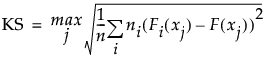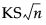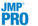Basic Analysis > Oneway Analysis > Nonparametric Tests > Kolmogorov-Smirnov Two-Sample Test Report
Publication date: 07/30/2020

## Kolmogorov-Smirnov Two-Sample Test Report

The Kolmogorov-Smirnov test is available only when X has exactly two levels. The report shows descriptive statistics followed by test results. The descriptive statistics are the following:

Level

The two levels of X.

Count

The frequencies of each level.

EDF at Maximum

For a level of X, gives the value of the empirical cumulative distribution function (EDF) for that level at the value of X for which the difference between the two EDFs is a maximum. For the row named Total, gives the value of the pooled EDF (the EDF for the entire data set) at the value of X for which the difference between the two EDFs is a maximum.

Deviation from Mean at Maximum

For each level, gives the value obtained as follows:

Compute the difference between the EDF at Maximum for the given level and the EDF at maximum for the pooled data set (Total).

Multiply this difference by the square root of the number of observations in that level, given as Count.

### Kolmogorov-Smirnov Asymptotic Test

This report gives the details for the test.

KS

A Kolmogorov-Smirnov statistic computed as follows:The formula uses the following notation:

xj, j = 1,..., n are the observations

ni is the number of observations in the ith level of X

F is the pooled cumulative empirical distribution function

Fi is the cumulative empirical distribution function for the ith level of X

This version of the Kolmogorov-Smirnov statistic applies even when there are more than two levels of X. Note, however, that JMP performs the Kolmogorov-Smirnov analysis only when X has only two levels of X.

KSa

An asymptotic Kolmogorov-Smirnov statistic computed as, where n is the total number of observations.

D=max|F1-F2|

The maximum absolute deviation between the EDFs for the two levels. This is the version of the Kolmogorov-Smirnov statistic typically used to compare two samples.

Prob > D

The p-value for the test. This is the probability that D exceeds the computed value under the null hypothesis of no difference between the levels.

D+ = max(F1-F2)

A one-sided test statistic for the alternative hypothesis that the level of the first group exceeds the level of the second group.

Prob > D+

The p-value for the test of D+.

D- = max(F2-F1)

A one-sided test statistic for the alternative hypothesis that the level of the second group exceeds the level of the first group

Prob > D-

The p-value for the test of D-.

###Kolmogorov-Smirnov Exact Test

For the Kolmogorov-Smirnov exact test, the report gives the same statistics as does the asymptotic test, but the p-values are computed to be exact.